Function Repository Resource:

# FrenetSerretPlot

Plot the Frenet–Serret frame of a curve

Contributed by: Wolfram Staff (original content by Alfred Gray)
 ResourceFunction["FrenetSerretPlot"][c,t0,{t,tmin,tmax}] plots the curve c with parameter t from tmin to tmax, with the Frenet–Serret frame for c at t0.

## Details and Options

ResourceFunction["FrenetSerretPlot"] has the same options as ParametricPlot3D.

## Examples

### Basic Examples (2)

A figure eight:

 In:=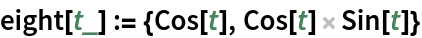The Frenet–Serret frame:

 In:=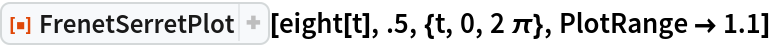Out=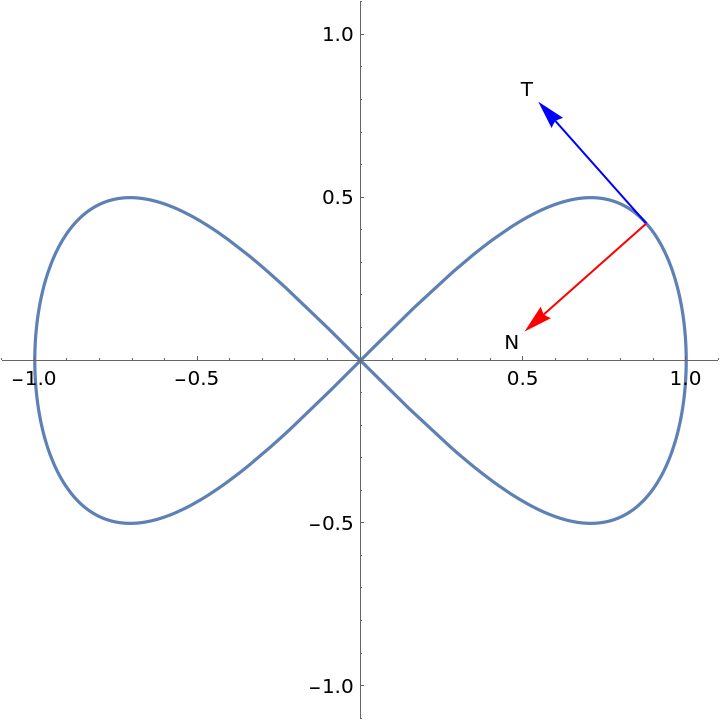See how the system changes as t0 is varied:

 In:=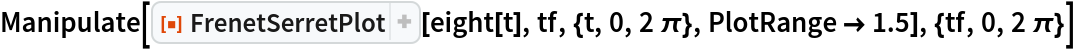Out=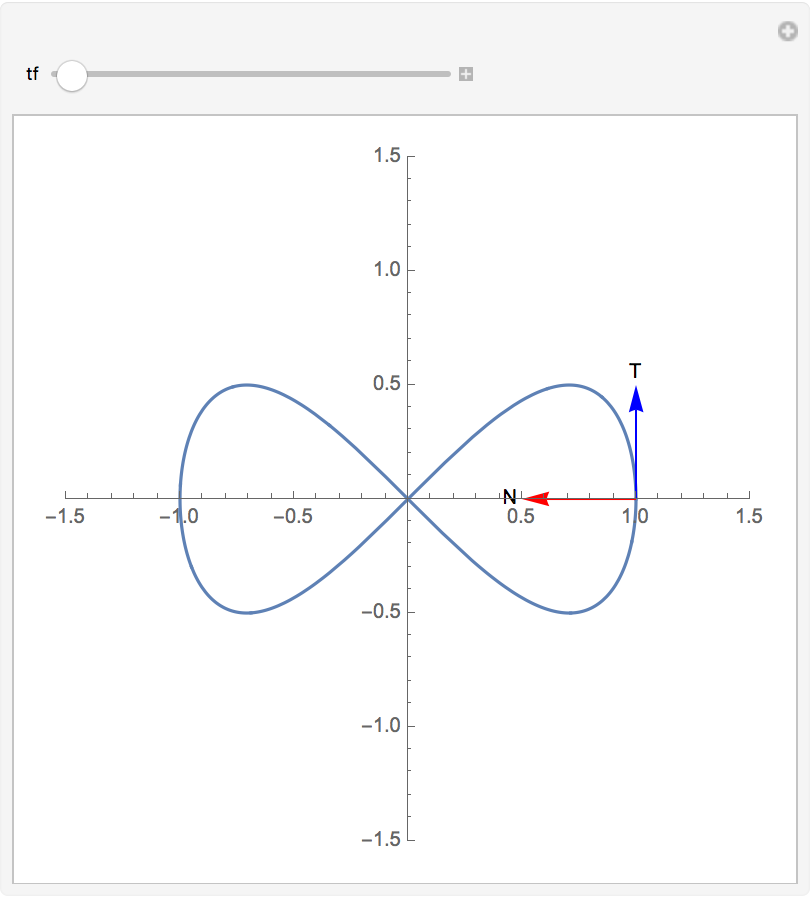Viviani’s curve:

 In:=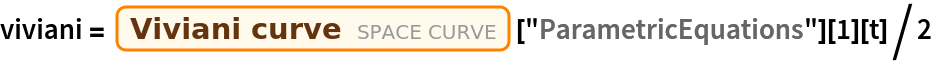Out=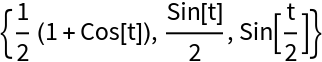The Viviani’s curve with an animation of the tangent, normal, and binormal moves along the curve:

 In:=Out=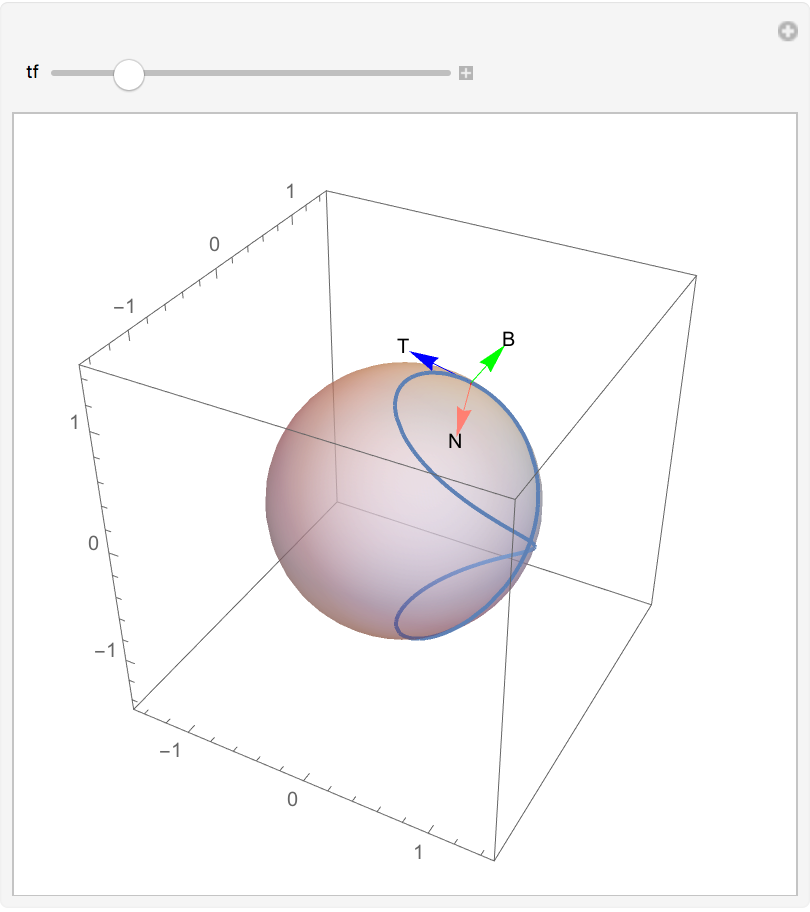### Options (4)

#### ScaleFactor (1)

Modify the length of vectors:

 In:=Out=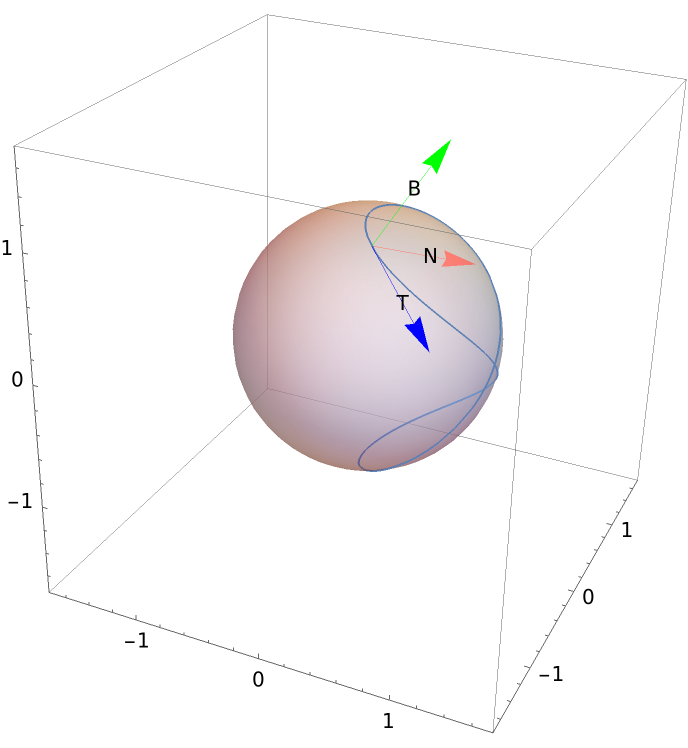#### FrameStyle (1)

Change the style of vectors:

 In:=Out=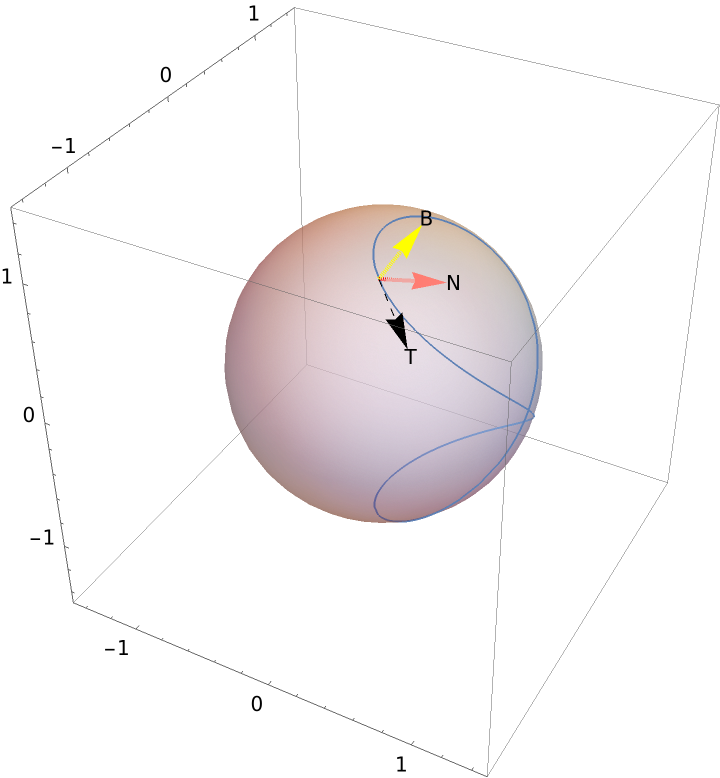#### FrameText (1)

Change the text for vectors of the frame:

 In:=Out=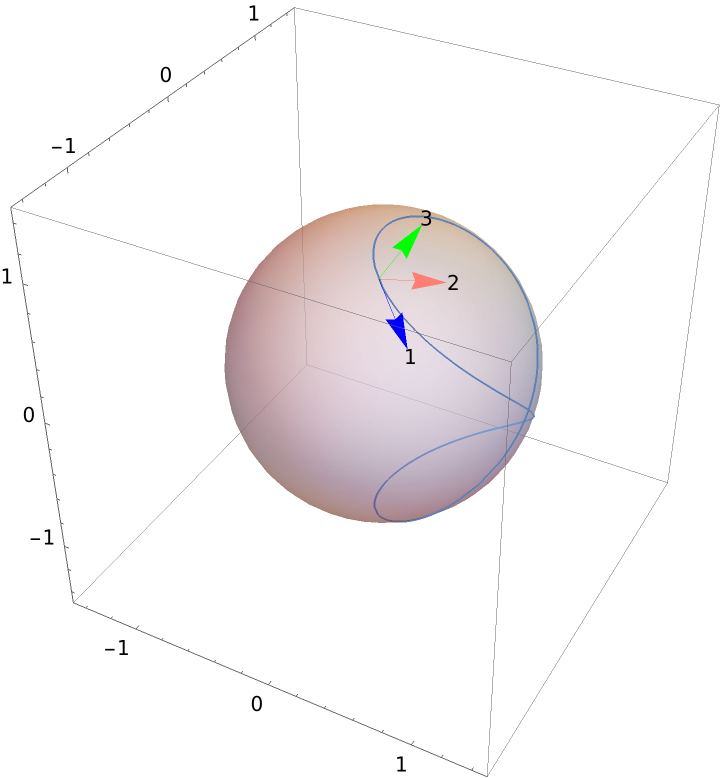#### FrameTextPosition (1)

 In:=Out=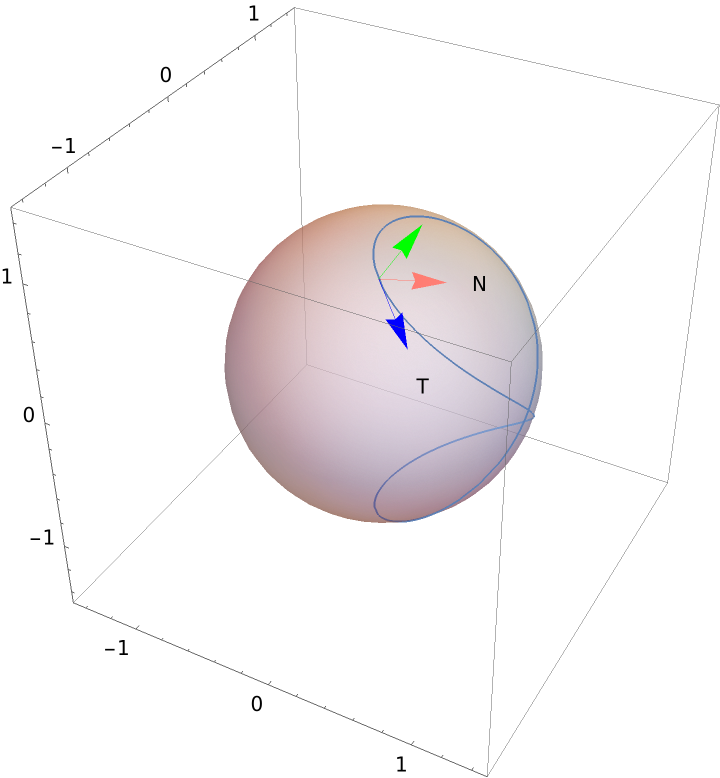Enrique Zeleny

## Version History

• 1.0.0 – 11 November 2020